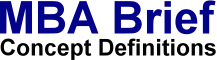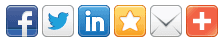# Hypothesis TestingDefinition: Hypothesis Testing is a statistical method of reasoning and decision making using data from a scientific study. The question of interest is simplified into two competing claims / hypotheses between which we have a choice; the null hypothesis (H0) against the alternative hypothesis (H1). These two competing claims / hypotheses are not however treated on an equal basis: special consideration is given to the null hypothesis. Statistical hypothesis tests define a procedure which controls (fixes) the probability of incorrectly deciding that a default position (null hypothesis) is incorrect based on how likely it would be for a set of observations to occur if the null hypothesis were true.

 More on hypothesis testing. More on problem solving: Catch-22, Evaporating Cloud, Heuristics, Intuition, Kepner-Tregoe, more...

© 2019 MBA Brief - Last updated: 21-7-2019  -  Privacy   |   Terms